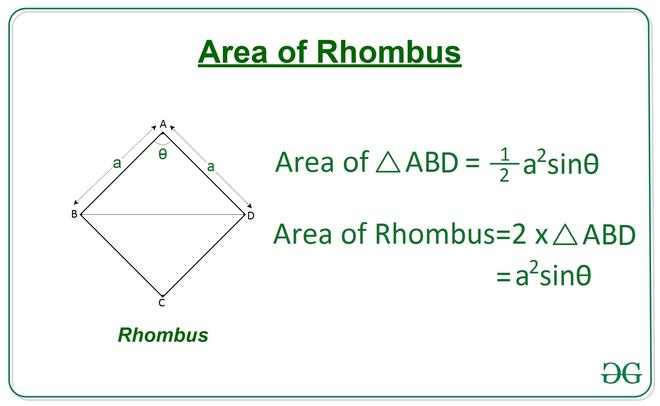# Find the area of rhombus from given Angle and Side length

• Last Updated : 07 Apr, 2021

Given two integers A and X, denoting the length of a side of a rhombus and an angle respectively, the task is to find the area of the rhombus.

A rhombus is a quadrilateral having 4 sides of equal length, in which both the opposite sides are parallel, and opposite angles are equal.

Examples:

Input: A = 4, X = 60
Output: 13.86

Input: A = 4, X = 30
Output: 8.0

Approach:For a Rhombus ABCD having the length of a side a and an angle x, the area of triangle ABD can be calculated using Side-Angle-Side property of triangle by the following equation:

Area of Triangle ABD = 1/2 (a2) sin x
Area of Rhombus ABCD will be double the area of ABD triangle.

Therefore, Area of Rhombus ABCD = (a2) sin xBelow is the implementation of the above approach:

## C++

 `// C++ Program to calculate` `// area of rhombus from given` `// angle and side length` `#include ` `using` `namespace` `std;`   `#define RADIAN 0.01745329252` `// Function to return the area of rhombus` `// using one angle and side.` `float` `Area_of_Rhombus(``int` `a, ``int` `theta)` `{` `    ``float` `area = (a * a) * ``sin``((RADIAN * theta));` `    ``return` `area;` `}`   `// Driver Code` `int` `main()` `{` `    ``int` `a = 4;` `    ``int` `theta = 60;`   `    ``// Function Call` `    ``float` `ans = Area_of_Rhombus(a, theta);`   `    ``// Print the final answer` `    ``printf``(``"%0.2f"``, ans);` `    ``return` `0;` `}`   `// This code is contributed by Rajput-Ji`

## Java

 `// Java Program to calculate` `// area of rhombus from given` `// angle and side length` `class` `GFG{`   `static` `final` `double` `RADIAN = ``0.01745329252``;` `  `  `// Function to return the area of rhombus` `// using one angle and side.` `static` `double` `Area_of_Rhombus(``int` `a, ``int` `theta)` `{` `    ``double` `area = (a * a) * Math.sin((RADIAN * theta));` `    ``return` `area;` `}`   `// Driver Code` `public` `static` `void` `main(String[] args)` `{` `    ``int` `a = ``4``;` `    ``int` `theta = ``60``;`   `    ``// Function Call` `    ``double` `ans = Area_of_Rhombus(a, theta);`   `    ``// Print the final answer` `    ``System.out.printf(``"%.2f"``, ans);` `}` `}`   `// This code is contributed by Rajput-Ji`

## Python3

 `# Python3 Program to calculate ` `# area of rhombus from given ` `# angle and side length` `  `  `import` `math  ` `  `  `# Function to return the area of rhombus ` `# using one angle and side.  ` `def` `Area_of_Rhombus(a, theta):  ` `  `  `    ``area ``=` `(a``*``*``2``) ``*` `math.sin(math.radians(theta))` `  `  `    ``return` `area  ` `  `  `# Driver Code  ` `a ``=` `4` `theta ``=` `60` `  `  `# Function Call  ` `ans ``=` `Area_of_Rhombus(a, theta)  ` `  `  `# Print the final answer` `print``(``round``(ans, ``2``)) `

## C#

 `// C# Program to calculate` `// area of rhombus from given` `// angle and side length` `using` `System;` `class` `GFG{`   `static` `readonly` `double` `RADIAN = 0.01745329252;` `  `  `// Function to return the area of rhombus` `// using one angle and side.` `static` `double` `Area_of_Rhombus(``int` `a, ``int` `theta)` `{` `    ``double` `area = (a * a) * Math.Sin((RADIAN * theta));` `    ``return` `area;` `}`   `// Driver Code` `public` `static` `void` `Main(String[] args)` `{` `    ``int` `a = 4;` `    ``int` `theta = 60;`   `    ``// Function Call` `    ``double` `ans = Area_of_Rhombus(a, theta);`   `    ``// Print the readonly answer` `    ``Console.Write(``"{0:F2}"``, ans);` `}` `}`   `// This code is contributed by Rajput-Ji`

## Javascript

 ``

Output:

`13.86`

Time Complexity: O(1)
Auxiliary Space: O(1)

My Personal Notes arrow_drop_up
Recommended Articles
Page :